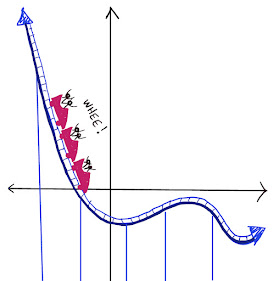## Thursday, March 10, 2022

### Same Sum Circles, Part 2

The goal of this puzzle is to place the numbers 1 through 7 in the circles so that you get the same sum in each circle.

Notice that the placement below is ALMOST a solution but not quite.  One circle has a sum of 17 and the other two have a sum of 19.  You can fix it by moving only one bean.  Which one?  Where would you move it?

Once you figure that out, see how many different sums you can make.  You can make quite a lot!

Click "read more" for the solution:
Solution:

You can solve the puzzle by moving the 2 into the same region as the 5.  That way, one circle still has a sum of 17 and the others are made smaller by 2.

Here are some other sums: